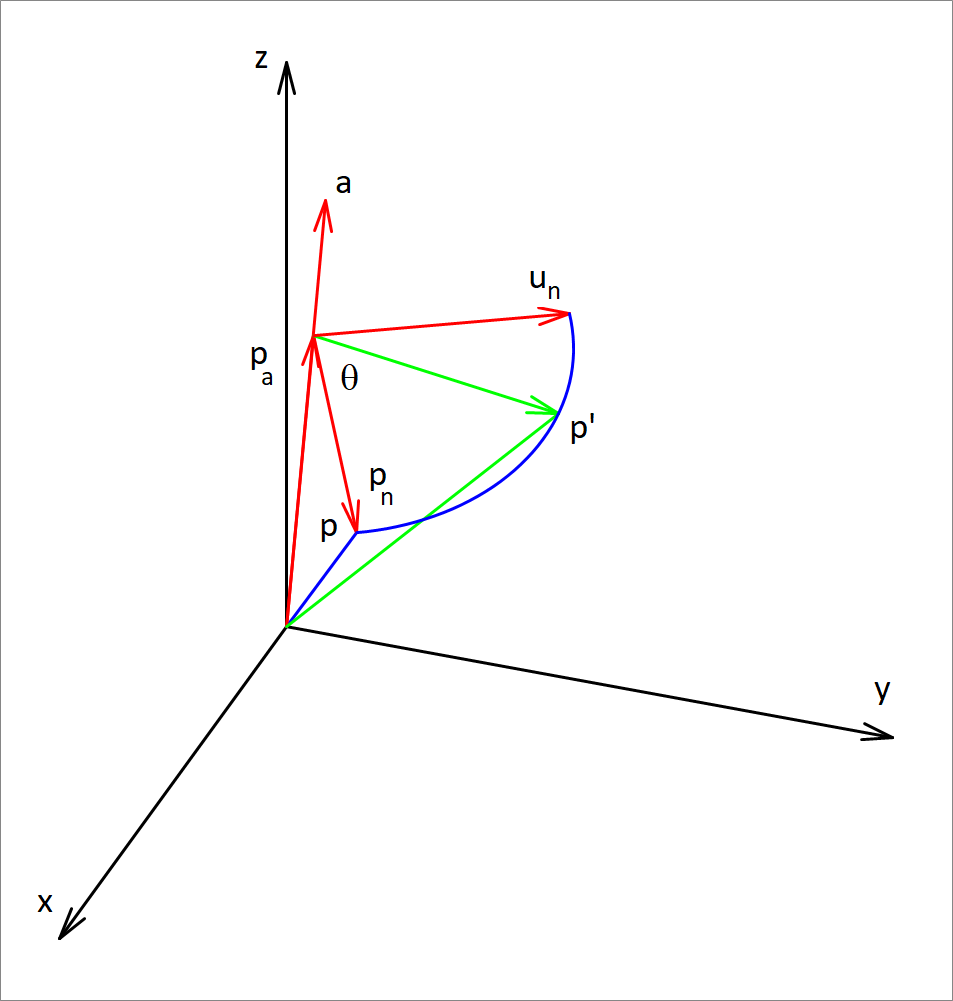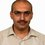# Rotation in 3D - The Rotation MatrixIn this note, I investigate the rotation matrix that relates the image of a point $\vec{p}$ when it is rotated by an angle $\theta$ about an axis $\vec{a}$ that passes through the origin.

Without loss of generality, we assume that the direction vector of the axis is a unit vector.

Now we decompose the vector $\vec{p}$, into two components, one along the rotation axis, and one orthogonal to it.

The component along the rotation axis is given by

$\vec{p_a} = ( \vec{p} . \vec{a} ) \vec{a}$

(remember that $\vec{a}$ is a unit vector so, $\vec{a} \cdot \vec{a} = 1$

And, the orthogonal component is,

$\vec{p_n} = \vec{p} - \vec{p_a} = \vec{p} - ( \vec{p} \cdot \vec{a} ) \vec{a}$

In matrix-vector notation, if all vectors are (3 x 1) column vectors, then

$\vec{p_a} = (\vec{p} \cdot \vec{a}) \vec{a} = (\vec{a} \cdot \vec{p}) \vec{a} = (\textbf{a}^T \textbf{p}) \textbf{a} = \textbf{a} (\textbf{a}^T \textbf{p}) = (\textbf{a} \textbf{a}^T) \textbf{p}$

and

$\vec{p_n} = \vec{p} - \vec{p_a} = ( \textbf{I} - \textbf{a} \textbf{a}^T ) \textbf{p}$

Now when the component $\textbf{p}_a$ undergoes rotation, it is unchanged.

It is $\textbf{p}_n$ that gets rotated about the axis $\textbf{a}$. To write an expression for the image of $\textbf{p}_n$ undergoing a rotation by $\theta$ , we need the perpendicular vector to both $\textbf{a}$ and $\textbf{p}_n$, and is at $90$ degrees counter-clockwise from $\textbf{p}_n$. This vector is

$\vec{u_n} = \vec{a} \times \vec{p_n} = \vec{a} \times (\vec{p} - \vec{p_a}) = \vec{a} \times \vec{p}$

because $\vec{a} \times \vec{p_a} = 0$ since the two vectors are collinear.

Noe that $|| \vec{u_n} || = || \vec{a} \times \vec{p_n} || = || \vec{p_n} ||$ because $\vec{a}$ is orthogonal to $\vec{p_n}$ and $\vec{a}$ is a unit vector.

So now the plane of rotation is spanned by the vectors $\vec{p_n}$ and $\vec{u_n}$, both equal in length , and that length is $|| \vec{p_n} ||$

With that in mind, and since the point describes a circle in the plane of rotation, then

$\vec{p_n} ' = \cos(\theta) \vec{p_n} + \sin(\theta) \vec{u_n}$

this expresses the rotation of the normal component of $\vec{p}$ around the axis $\vec{a}$.

Therefore, the rotated vector is

$\vec{p} ' = \vec{p_a} + \vec{p_n} ' = \vec{p_a} + \cos(\theta) \vec{p_n} + \sin(\theta) \vec{u_n} = \vec{p_a} + \cos(\theta) \vec{p_n} + \sin(\theta) (\vec{a} \times \vec{p} )$

Using the expressions derived above, we arrive at

$\textbf{p} ' = (\textbf{a} \textbf{a}^T) \textbf{p} + \cos(\theta) (\textbf{I} - \textbf{a} \textbf{a}^T) \textbf{p} + \sin(\theta) (\textbf{a} \times \textbf{p})$

Now,

$\textbf{a} \times \textbf{p} = \textbf{S}_a \textbf{p}$

where $\textbf{S}_a$ is a 3 x 3 skew-symmetric matrix given by

$\textbf{S}_a = \begin{bmatrix} 0 & -a_z & a_y \\ a_z & 0 & -a_x \\ -a_y & a_x & 0 \end{bmatrix}$

(that is, the vector cross product $\textbf{a} \times \textbf{p}$ , can be expressed as a matrix-vector multiplication)

and hence, finally,

$\textbf{p}' = \textbf{R} \textbf{p}$

where

$\textbf {R} = \textbf{a} \textbf{a}^T + \cos(\theta) ( I - \textbf{a} \textbf{a}^T ) + \sin(\theta) \textbf{S}_a$

is the desired rotation matrix.

What if the axis of rotation did not pass through the origin, but passed though a point $\textbf{p}_0 = [x_0, y_0, z_0]$ ?

In this case, we can translate (shift) the origin of the reference frame to the point $\textbf{p}_0$. Since $\textbf{p}_0$ is on the axis of rotation it is unaffected by the rotation, and its image is itself. Then, we apply our result to the vector $(\textbf{p} - \textbf{p}_0)$ which joins the axis of rotation at the point $\textbf{p}_0$.

Therefore,

$\textbf{p} ' = \textbf{p}_0 + \textbf{R} ( \textbf{p} - \textbf{p}_0)$Note by Hosam Hajjir
6 years, 8 months ago

This discussion board is a place to discuss our Daily Challenges and the math and science related to those challenges. Explanations are more than just a solution — they should explain the steps and thinking strategies that you used to obtain the solution. Comments should further the discussion of math and science.

When posting on Brilliant:

• Use the emojis to react to an explanation, whether you're congratulating a job well done , or just really confused .
• Ask specific questions about the challenge or the steps in somebody's explanation. Well-posed questions can add a lot to the discussion, but posting "I don't understand!" doesn't help anyone.
• Try to contribute something new to the discussion, whether it is an extension, generalization or other idea related to the challenge.

MarkdownAppears as
*italics* or _italics_ italics
**bold** or __bold__ bold
- bulleted- list
• bulleted
• list
1. numbered2. list
1. numbered
2. list
Note: you must add a full line of space before and after lists for them to show up correctly
paragraph 1paragraph 2

paragraph 1

paragraph 2

[example link](https://brilliant.org)example link
> This is a quote
This is a quote
    # I indented these lines
# 4 spaces, and now they show
# up as a code block.

print "hello world"
# I indented these lines
# 4 spaces, and now they show
# up as a code block.

print "hello world"
MathAppears as
Remember to wrap math in $$ ... $$ or $ ... $ to ensure proper formatting.
2 \times 3 $2 \times 3$
2^{34} $2^{34}$
a_{i-1} $a_{i-1}$
\frac{2}{3} $\frac{2}{3}$
\sqrt{2} $\sqrt{2}$
\sum_{i=1}^3 $\sum_{i=1}^3$
\sin \theta $\sin \theta$
\boxed{123} $\boxed{123}$

Sort by:

nice work! you have solved something which is easy to imagine hard to solve but i didn't get the matrix step how did you use the transpose part. i am a novice at linear algebra so please explain in little simpler terms

- 6 years, 3 months ago

I am sorry, I cannot make it any simpler. I suggest you wait till you've gained a working experience with Linear Algebra, then revisit this note, but thanks for the complement.

- 6 years, 3 months ago